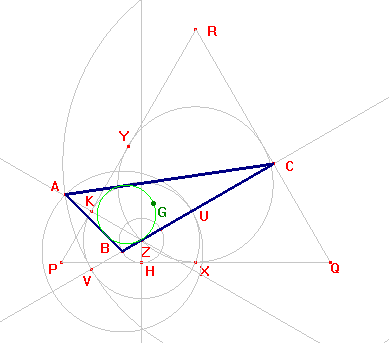c10

Triangle having its centroid at the incircle

1) Let PQR be an equilateral triangle. Draw its incircle.
2) Let X, Y be the contact points between it and the sides PQ, PR. Take Z in the lesser arc XY.
3) Let C, H, K be the orthogonal projections of Z on QR, PQ, PR.
4) Take B on CZ such as ZB = ZH.
5) The circle through K with center Z cuts CB at U, V (ordered C, U, Z, V).
6) Draw the circles with center B through U and with center C through V.
7) These circles intersect at A and ABC is the requested triangle.